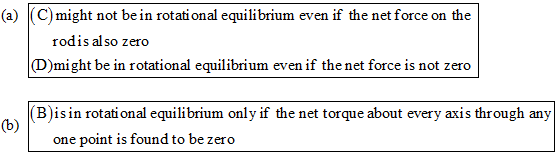×#### Thank you for registering.

One of our academic counsellors will contact you within 1 working day.

Click to Chat

1800-1023-196

+91-120-4616500

CART 0

• 0

MY CART (5)

Use Coupon: CART20 and get 20% off on all online Study Material

ITEM
DETAILS
MRP
DISCOUNT
FINAL PRICE
Total Price: Rs.

There are no items in this cart.
Continue Shopping
```
A long straight rod experiences several forces, each acting at a different location on the rod. All forces are perpendicular to the rod. The rod might be in translational equilibrium, rotational equilibrium, both, or neither. (a) If a calculation reveals that the net torque about the left end is zero, then one can conclude that the rod (A) is definitely in rotational equilibrium. (B) is in rotational equilibrium only if the net force on the rod is also zero. (C) might not be in rotational equilibrium even if the net force on the rod is also zero. (D) might be in rotational equilibrium even if the net force is not zero. (b) If a calculation reveals that the net force on the rod is zero, then one can conclude that the rod (A) is definitely in rotational equilibrium. (B) is in rotational equilibrium only if the net torque about every axis through anyone point is found to be zero. (C) might be in rotational equilibrium if the net torque about every axis through anyone point is found to be zero. (D) might be in rotational equilibrium even if the net torque about any axis through anyone point is not zero.

```
5 years agoNavjyot Kalra
654 Points
```							The correct option is:(a) When the calculations show that the net torque from the left end is zero, then it cannot be said certainly that the rod will be in rotational equilibrium. This will be possible only when the torque from the right end is also zero.It is possible for the rod to have a zero net force and still be rotating about the axis. Consider that a force passes through the axis of rotation of rod and one with same magnitude passes from the right, then there is a net torque on the rod but there is no net force on it.If the net force on the rod is zero, it might be that the rod is not in rotational equilibrium.Therefore (C) is the correct option.It is also possible that net force on the rod is not zero but the rod is still in rotational equilibrium. Consider that a force acts on the axis of rotation of the rod and two acts on the right side of the rod. The magnitude of the forces is equal, but the forces on the right side are opposite to each other. It is obvious that the force passing through the axis of rotation will be in phase with one of the force on the right side.Therefore the torque from the right side is equal, however there is a net force acting on the rod. It is important to note that the torque from the force acting at the axis of rotation will be zero, and has no turning effect.Therefore (D) is also the correct option(b) When the calculations reveal that there is no net force in the rod, it does not mean that the rod will be in rotational equilibrium. Consider for instance that a force 2F acts at the center of the rod and passing through the axis of rotation, and two other forces F (in same direction) acts on the right side of the rod. Then the rod will have net zero force but will still be in rotational equilibrium.If the torque about all the axes at a point is zero, then the rod will be in rotational equilibrium about that point.Therefore (B) is the correct option and rule out option (C) and option (D).
```
5 years ago
Think You Can Provide A Better Answer ?

## Other Related Questions on Mechanics

View all Questions »### Course Features

• 101 Video Lectures
• Revision Notes
• Previous Year Papers
• Mind Map
• Study Planner
• NCERT Solutions
• Discussion Forum
• Test paper with Video Solution### Course Features

• 110 Video Lectures
• Revision Notes
• Test paper with Video Solution
• Mind Map
• Study Planner
• NCERT Solutions
• Discussion Forum
• Previous Year Exam Questions